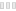CleanAlso available as portable version Advanced Trigonometry Calculator PortableA review by John

As much as mathematics revolves around four operations (addition, subtraction, division, and multiplication) there are other mathematical operations that need a special tool to simplify the process. This is an app that provides the scientific version of mathematical processes. The name confuses users to be just specifically for trigonometric calculations-no. It handles logarithms, hyperbolic operations among other mathematical concepts. This is a simple application that runs on both command line and automated syntax formulas. The command line comes in handy to manually input all the desired figures while the automated functions are predesigned formats that you need to select to complete a mathematical operation.

Some of the functions include quadratic equations, linear equations, geometry, linear programming among others. Despite the complex processes, you have a help file to aid you when stuck in running any of the operations. In fact, you can restart the app and even reset them to its default position or even save what you have done for further use. The latter is ideal when handling statistical operations. If you are tired of manually handling numbers from the simple arithmetic calculations to the complex ones then you now have a tool to make handling figures easy and fast.

Advanced Trigonometry Calculator is licensed as freeware for PC or laptop with Windows 32 bit and 64 bit operating system. It is in mathematics category and is available to all software users as a free download.

 Share Give a rating(0 votes, average: 0.00 out of 5)login to voteLoading... Author Renato Alexandre Santos Freitas Last Updated On August 30, 2019 Runs on Windows 10 / Windows 8 / Windows 7 / Windows Vista / XP Total downloads 217 License Free File size 2,39 MB Filename Setup x86.exeSetup x64.exe

### Similar programsGraphing Calculator 3D
Plot math equations, graphs in 2D / 3D
FreeGreat Stella
Create multiple polyhedral objectsMATLAB
Tool for math calculationsMath Mechanixs